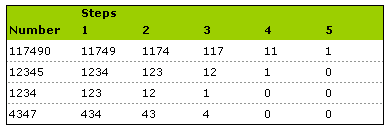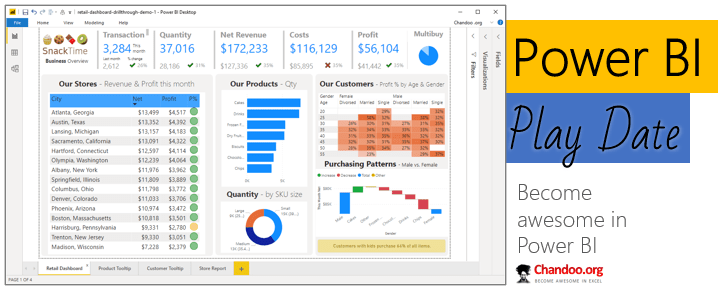# Seperating digits from a number [excel formulas]

Share

Take the number 117490. I need to break it down5 times:

11749
1174
117
11
1

You can use FLOOR() function to get the desired result, like this:
`=FLOOR(your-number/10^digit-no,1)`

See the image below:### Get FREE Excel + Power BI Tips

Simple, fun and useful emails, once per week.

Learn & be awesome.

### Welcome to Chandoo.org

Thank you so much for visiting. My aim is to make you awesome in Excel & Power BI. I do this by sharing videos, tips, examples and downloads on this website. There are more than 1,000 pages with all things Excel, Power BI, Dashboards & VBA here. Go ahead and spend few minutes to be AWESOME.

Read my storyFREE Excel tips book

Chandoo is an awesome teacher
5/5

– Jason

Still on fence about Power BI? In this getting started guide, learn what is Power BI, how to get it and how to create your first report from scratch.### Introducing Power BI Play Date – Online class to master Power BI (oh yeah, Power Query & Power Pivot too)

Hello friends,

Check out below slideshow to see some of the reports you can create in our course. It is #AWESOME.

## Related Tips

### 17 Responses to “Seperating digits from a number [excel formulas]”

1.Joann Lindenschmidt says:

My state tax dept requires a "code" for payment amounts which is the sum of the digits for the payment plus the number of digits.
Example: \$1045.99 = code 34
1 + 0 + 4 + 5 + 9 + 9 plus 6 (digits in my pymt amt)

Would the floor() function do that?

Also, I would never have known what the floor() function would do. Is there some type of function definition list somewhere?

2.FMulvaney says:

@Joann Lindenschmidt
Hello Joann. I'm a new reader on this great site. Maybe I can help you. The Floor function rounds a number down to the nearest multiple of significance you specify. For example if you take 2.5 and specify 1 as the multiple the result would be 2. In the example above the Floor function eliminates noise in the formula. One way to see functions and to get help with them is by clicking on the fx (insert function) button on the left of the formula bar. You can type in a function or select all and click through each function to see what they do. You can also let the insert function dialogue box walk you through setting up a function. Its very useful when you are new to functions.

The Left function might also be used to seperate digits from a number. In the example above Left(117490,4) would return 1174.

I don't think the Floor function would help you in the example you posted but I may be missing something.

3.Chandoo says:

@FMulvaney: Welcome 🙂 thanks for answering the questions. I am sure Joann would appreciate it.

@Joann: For summing individual digits and adding the length you can use a formula like mid() which extracts a part of cell's content based on starting point and length you specify.

For eg. mid("117490",2,1) would return 1

But, if you are looking for a single cell formula, here is one I could think of. It is little lengthy and difficult to maintain but works like charm.

=1*(LEN(\$B\$33)-LEN(SUBSTITUTE(\$B\$33,"1","")))+2*(LEN(\$B\$33)-LEN(SUBSTITUTE(\$B\$33,"2","")))+3*(LEN(\$B\$33)-LEN(SUBSTITUTE(\$B\$33,"3","")))+4*(LEN(\$B\$33)-LEN(SUBSTITUTE(\$B\$33,"4","")))+5*(LEN(\$B\$33)-LEN(SUBSTITUTE(\$B\$33,"5","")))+6*(LEN(\$B\$33)-LEN(SUBSTITUTE(\$B\$33,"6","")))+7*(LEN(\$B\$33)-LEN(SUBSTITUTE(\$B\$33,"7","")))+8*(LEN(\$B\$33)-LEN(SUBSTITUTE(\$B\$33,"8","")))+9*(LEN(\$B\$33)-LEN(SUBSTITUTE(\$B\$33,"9","")))+LEN(\$B\$33)

just change \$b\$33 to your cell with payments.

4.derek says:

Here's a challenge I got from work: a colleague needs to visually extract a number from a longer string, and wanted a way that was less eye-straining. The number is less than 10.0 (ie., only one digit in front of the decimal), and may have between one and four digits after the decimal. There is a space before and a space after, but all sorts of things can come in the string as well. How can I reliably extract that number from the junk?

My solution, IIRC, was

=MID(A1&REPT(" ",12),(SEARCH(A1,"?.????"))-3,12)

and I conditionally formatted to de-emphasise the #VALUE! that shows up when the number is absent or undetected. Including the three characters on either side of the number helps in visually confirming that the detection was accurate, and the twelve appended spaces avoid errors caused by the number being too close to the end of the original string.

Any improvements?

5.derek says:

Rats. Make that ” ?.????”. I include the leading space for the detection. as it is the only reliable element, besides the decimal point. If only Excel had regexp so I could instruct it to look for digits!

6.Robert says:

Derek,

here is a more general, array-based approach (Text is in cell A1):

=MID(LEFT(A1,MAX(IF(ISNUMBER(MID(A1,COLUMN(1:1),1)*1),COLUMN(1:1)))),MATCH(TRUE,ISNUMBER(MID(A1,COLUMNS(1:1),1)*1),0),LEN(A1))

1. Handles all options (number at the beginning, at the end, in the middle)
2. Works for all numbers regardless the number of digits
3. Works for dates and times as well

Shortfalls:

1. It is an array formula and we all know about the advantages and disadvantages of array formulas.

2. Returns an error if the text does not include a number. IF (ISERROR(formula),””,formula) can only be used in Excel 2007. Excel 2003 and earlier has a limitation of nested IFs (I think maximum 7), therefore you have to use user-defined formats or an extra help column for the error handling.

3. It does not handle negative values (‘-‘ is treated as a character and therefore cut off). The formula always brings back the positive value.

4. It does not work properly for texts with more than one number. It will bring back a string with everything between the first digit of the first number and the last digit of the last number.

5. You might run into a problem with the different numbering formats in different countries. E.g. in the U.S. you are using ‘,’ as the thousand separator and ‘.’ as the decimal point. In Europe it is exactly the other way round.

7.Robert says:

O.k, this formula is obviously too long for a WordPress comment. Here it is again:

=MID(

LEFT(A1,MAX(IF(ISNUMBER(MID(A1,COLUMN(1:1),1)*1),COLUMN(1:1)))),

MATCH(TRUE,ISNUMBER(MID(A1,COLUMNS(1:1),1)*1),0),

LEN(A1))

As said above an array formula, insert it with ctrl-shift-return.

8.Ketan says:

@Joann ,Chandoo :

Cud u pls make cusotmise function in VBA by using the following in loop :
1)function "len"
2)function "mid"
This function can be used in any cell.

Logic :
Say the name of function PHDC ( )
Use counter and make a loop for "No. of Characters" (by "len" function)
Use "mid" function and store the value of each loop
Close the loop till counter reach to "No. of Characters"
The final formula in result cell will be =PHDC( ) + len ( )
Just make and inform

9.Chandoo says:

@Derek: .. I couldn't think of a better way to write this formula without increasing its length. I guess your version is working fine and since you are using conditional formatting to de-emphasize errors there is probably no need to wrap it either.

@Robert: awesome stuff... 🙂

10.Chandoo says:

@Ketan: Good suggestion. Even though VBA remains a powerful tool to automate several things, most of us consciously try to avoid it in day to day work. Also, I make sure to use non-vba solutions whenever I can because they are easy to learn and use.

11.derek says:

I liked Robert's suggestion, and I'll try it out later this week if I get time.

12.pradeep says:

hi, please somebody help me to solve my problem as bellow:
i have a double digit in single cell = 12
i need the sum of that digit =3

13.Chandoo says:

@Pradeep: There is a very good array formula that can do this, http://support.microsoft.com/kb/214053

Also, if you know that your data has only 2 digits, then you can use this simpler formula: (assuming data is in cell B2)

=int(c2/10)+mod(c2,10)

14.hemant says:

i have decimal numbers in the cell separated by backslash. i want each of this decimal numbers to be separated in different cells.

eg: if say number in a cell A1 is 22.1/12.1/2.15/3

the output in cells A2, A3, A4 and A5 should be 22.1, 12.1, 2.15 and 3 respectively.

•mani says:

hii friends,
now, i want to do sum of this number 9846625248, but i have done like this =9+8+4+6+6+2+5+2+4+8, but how to do the sum of this number by using a excel formula? so is anyboday explain with formula and example.

regards
mani

15.Sonam says:

I want to round off a number with its ones place digit.
Number typed like this but show like this
23654 23655
23648 23650
5621 5625
3265 3625
466 470
14969 14970

### Get FREE Excel & Power-BI Newsletter

One email per week with Excel and Power BI goodness. Join 100,000+ others and get it free.